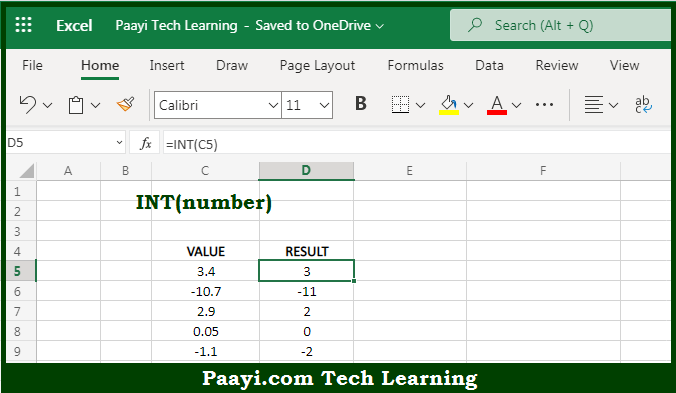# Learn How to Use Microsoft Excel INT Function

Written by | 0 Comments | 651 Views

In this article, you will learn how to use the Microsoft Excel INT function and its prime function in Microsoft Excel. You will also get to know the Microsoft Excel INT function return value and syntax with the help of some examples.

Microsoft Excel INT Function

The main function of the Microsoft Excel INT function is used to get the integer part of the decimal by rounding down. So, with the help of the INT function, you can able to return the integer part of the decimal by rounding it down to the integer. It should be noted that the INT s used to round down, so the negative number becomes more negative and the positive number becomes less positive. So, with the help of the Microsoft Excel INT function, you can easily able to get the integer part of the number by rounding down the number.

Return Value of INT Function

The return value will be the integer part of the number after rounding down.

Syntax of INT Function

=INT(number)

Where the arguments:

• number: This is the number of which you want to get an integer after rounding down.

## How to Use Microsoft Excel INT Function?So we know that Microsoft Excel INT function you can able to get the integer part of the decimal by rounding down. So, with the help of the INT function, you can able to return the integer part of the decimal by rounding it down to the integer. It should be noted that the INT s used to round down, so the negative number becomes more negative and the positive number becomes less positive. So, with the help of the Microsoft Excel INT function, you can easily able to get the integer part of the number by rounding down the number.